JEE  >  Competition Level Test: Continuity And Differentiability- 1

# Competition Level Test: Continuity And Differentiability- 1

Test Description

## 30 Questions MCQ Test Mathematics For JEE | Competition Level Test: Continuity And Differentiability- 1

Competition Level Test: Continuity And Differentiability- 1 for JEE 2023 is part of Mathematics For JEE preparation. The Competition Level Test: Continuity And Differentiability- 1 questions and answers have been prepared according to the JEE exam syllabus.The Competition Level Test: Continuity And Differentiability- 1 MCQs are made for JEE 2023 Exam. Find important definitions, questions, notes, meanings, examples, exercises, MCQs and online tests for Competition Level Test: Continuity And Differentiability- 1 below.
Solutions of Competition Level Test: Continuity And Differentiability- 1 questions in English are available as part of our Mathematics For JEE for JEE & Competition Level Test: Continuity And Differentiability- 1 solutions in Hindi for Mathematics For JEE course. Download more important topics, notes, lectures and mock test series for JEE Exam by signing up for free. Attempt Competition Level Test: Continuity And Differentiability- 1 | 30 questions in 60 minutes | Mock test for JEE preparation | Free important questions MCQ to study Mathematics For JEE for JEE Exam | Download free PDF with solutions
 1 Crore+ students have signed up on EduRev. Have you?
Competition Level Test: Continuity And Differentiability- 1 - Question 1

### A function f(x) is defined as below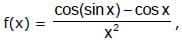x ≠ 0 and f(0) = a, f(x) is continuous at x = 0 if a equals

Competition Level Test: Continuity And Differentiability- 1 - Question 2

###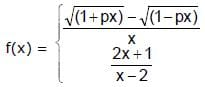, -1 ≤ x < 0 is continuous in the interval [–1, 1], then `p' is equal to:

Detailed Solution for Competition Level Test: Continuity And Differentiability- 1 - Question 2

lim f(x) = -½
Apply L hospital
lim(x → 0) p/2(1+px)½ + p/2(1-px)½ = -½
⇒ 2p = -1
p = -1/2

Competition Level Test: Continuity And Differentiability- 1 - Question 3

### Let f(x) =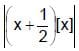when – 2 ≤ x ≤ 2. Then (where [ * ] represents greatest integer function)

Detailed Solution for Competition Level Test: Continuity And Differentiability- 1 - Question 3

At (x = 0) f(0) = |(0+½) .| = 0
f(1) = |(1+½) .| = 3/2
f(2) = |(2+½) .| = 5
f(-1) = |(-1+½) .[-1]| = ½
LHL at x = 2
lim x-->2  f(x) = |(x+½) .|
=|(5/2) * 1| = 5/2
f(2) = limx-->2 f(x) ( continuous at x=2)

Competition Level Test: Continuity And Differentiability- 1 - Question 4

Let f(x) = sgn (x) and g(x) = x (x2 – 5x + 6). The function f(g(x)) is discontinuous at

Competition Level Test: Continuity And Differentiability- 1 - Question 5

If y =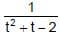where t =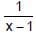, then the number of points of discontinuities of y = f(x), x ∈ R is

Detailed Solution for Competition Level Test: Continuity And Differentiability- 1 - Question 5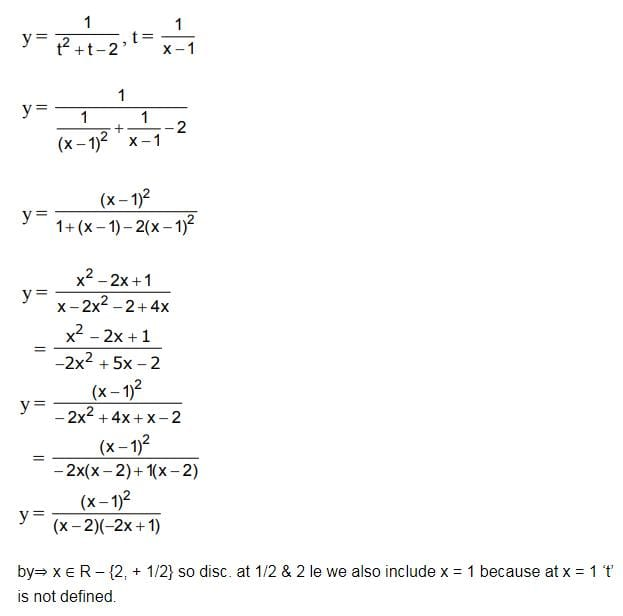Competition Level Test: Continuity And Differentiability- 1 - Question 6

The equation 2 tan x + 5x – 2 = 0 has

Detailed Solution for Competition Level Test: Continuity And Differentiability- 1 - Question 6

Let f(x) = 2tan x + 5x – 2
Now, f(0) = – 2 < 0
and f(π/4 ) = 2 + 5π/4 – 2
= 5π/5 > 0,
the line intersects the x-axis at x = ⅖
since 0 < ⅖ <  π/4
Thus, f(x) = 0 has atleast real solution root in ( 0, π/4)

Competition Level Test: Continuity And Differentiability- 1 - Question 7

If f(x) =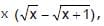, then indicate the correct alternative(s)

Competition Level Test: Continuity And Differentiability- 1 - Question 8

The function f (x) = 1 + | sin x l is

Competition Level Test: Continuity And Differentiability- 1 - Question 9

If f(x) =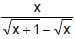be a real valued function then

Competition Level Test: Continuity And Differentiability- 1 - Question 10

The function f(x) = sin-1 (cos x) is

Competition Level Test: Continuity And Differentiability- 1 - Question 11

Let f(x) be defined in [–2, 2] by f(x) =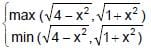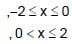then f(x)

Competition Level Test: Continuity And Differentiability- 1 - Question 12

If f(x) is differentiable everywhere, then

Detailed Solution for Competition Level Test: Continuity And Differentiability- 1 - Question 12

Even if f(x) is differentiable everywhere, |f(x)| need not be differentiable everywhere. An example being where the function changes its sign from negative to positive. f(x)=x at x=0B.
∣f∣= {f if f>0                −f if f<0}
​So,∣f∣2 = {f2 if f>0        −f2 if f<0}
​Differentiating ∣f∣2,
{2ff′ if f>0     −2ff′ if f<0}
​At f=0, LHD=RHD=∣f(0)∣2=0, so function is differentiable.

Competition Level Test: Continuity And Differentiability- 1 - Question 13

Let f(x + y) = f(x) f(y) all x and y. Suppose that f(3) = 3 and f'(0) = 11 then f'(3) is given by

Detailed Solution for Competition Level Test: Continuity And Differentiability- 1 - Question 13

f(x + y)=f(x) f(y) wrt x we get:
f′(x+y) (1 + y′) = f(x) f′(y) (y′) + f′(x) f(y)
Now on substituting x = 0 and y = 3, we get :
f′(3) (1 + 0)=f(0) f′(3) 0+ f(3) f′(0)
f′(3) = 3 × 11 = 33

Competition Level Test: Continuity And Differentiability- 1 - Question 14

If f : R → R be a differentiable function, such that f(x + 2y) = f(x) + f(2y) + 4xy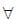x, y ∈ R, then

Competition Level Test: Continuity And Differentiability- 1 - Question 15

Let f(x) = x – x2 and g(x) =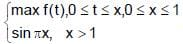. Then in the interval [0, ¥)

Competition Level Test: Continuity And Differentiability- 1 - Question 16

Let [x] denote the integral part of x ∈ R and g(x) = x – [x]. Let f(x) be any continuous function with f(0) = f(1) then the function h(x) = f(g(x))

Competition Level Test: Continuity And Differentiability- 1 - Question 17

If f (x) = [x sin p x] { where [x] denotes greatest integer function}, then f (x) is

Competition Level Test: Continuity And Differentiability- 1 - Question 18

If f(x) =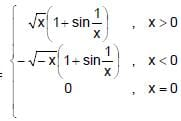, then f(x) is

Competition Level Test: Continuity And Differentiability- 1 - Question 19

The functions defined by f(x) = max {x2, (x – 1)2, 2x (1 – x)}, 0 £ x £ 1

Competition Level Test: Continuity And Differentiability- 1 - Question 20

Let f(x) = x3 – x2 + x + 1 and g(x) =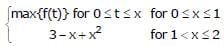then

Competition Level Test: Continuity And Differentiability- 1 - Question 21

Let f²(x) be continuous at x = 0 and f²(0) = 4 then value of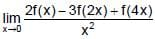is

Competition Level Test: Continuity And Differentiability- 1 - Question 22

Let f : R → R be a function such that f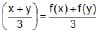, f(0) = 0 and f¢(0) = 3, then

Competition Level Test: Continuity And Differentiability- 1 - Question 23

Suppose that f is a differentiable function with the property that f(x + y) = f(x) + f(y) + xy and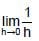f(h) = 3 then

Competition Level Test: Continuity And Differentiability- 1 - Question 24

If a differentiable function f satisfies f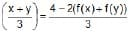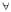x, y Î R, find f(x)

Competition Level Test: Continuity And Differentiability- 1 - Question 25

Let f : R → R be a function defined by f(x) = Min {x + 1, |x| + 1}. Then which of the following is true ?

Detailed Solution for Competition Level Test: Continuity And Differentiability- 1 - Question 25

f(x) = Min {x + 1,∣x∣ + 1}
x = 0 ⇒ ∣x∣ = x
f(x) = min {x + 1,x + 1}
= x + 1
x < 0,∣x∣ = −x
f(x) = min {x + 1,−x + 1}
−x + 1> x + 1
x < 0
⇒x < 0, f(x) = x + 1
x > 0,f (x) = x + 1
⇒ f(x) = x + 1 for all x
∴f(x) is differential everywhere (continuous and constant slope)

Competition Level Test: Continuity And Differentiability- 1 - Question 26

The function f : R /{0} → R given by vf(x) =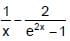can be made continuous at x = 0 by defining f(0) as

Detailed Solution for Competition Level Test: Continuity And Differentiability- 1 - Question 26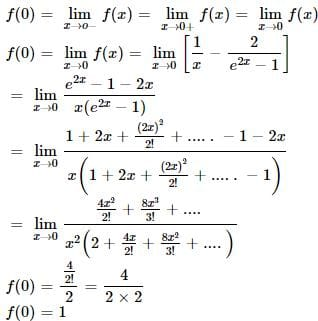Competition Level Test: Continuity And Differentiability- 1 - Question 27

Function f(x) = (|x – 1| + |x – 2| + cos x) where x Î [0, 4] is not continuous at number of points

Competition Level Test: Continuity And Differentiability- 1 - Question 28

Let f(x + y) = f(x) f(y) for all x, y, where f(0) ≠ 0. If f'(0) = 2, then f(x) is equal to

Competition Level Test: Continuity And Differentiability- 1 - Question 29

A function f : R → R satisfies the equation f(x + y) = f(x) . f(y) for all x, y ∈ R, f(x) ≠ 0. Suppose that the function is differentiable at x = 0 and f'(0) = 2 then f'(x) =

Competition Level Test: Continuity And Differentiability- 1 - Question 30

Let f(x) = [cos x + sin x], 0 < x < 2p where [x] denotes the greatest integer less than or equal to x. the number of points of discontinuity of f(x) is

## Mathematics For JEE

130 videos|359 docs|306 tests
Information about Competition Level Test: Continuity And Differentiability- 1 Page
In this test you can find the Exam questions for Competition Level Test: Continuity And Differentiability- 1 solved & explained in the simplest way possible. Besides giving Questions and answers for Competition Level Test: Continuity And Differentiability- 1, EduRev gives you an ample number of Online tests for practice

## Mathematics For JEE

130 videos|359 docs|306 tests(Scan QR code)